# Theoretical background¶

## eDisGo Cluster Method¶

In order to achieve acceptable computation times, the problem’s complexity can be reduced by applying a k-means cluster-algorithm to MV grids. The algorithm identifies a specified number of representative MV grids and assigns a weighting to each grid. As described here, the available clustering attributes are:

• cumulative installed wind capacity,
• cumulative installed solar capacity,
• distance between transition point and farthest node of the MV grid
• installed battery capacity (as a result of eTraGo’s investment optimization)

Subsequent to the MV grid simulations with the reduced number of representative grids, the cluster weighting is used to extrapolate the costs back to the original number of MV grids.

## Economic calculation¶

The tool eGo unites the extra high (ehv) and high voltage (hv) models with the medium (mv) and low voltage (lv) models to ascertain the costs per selected measure and scenario. This results in a cross-grid-level economic result of the electrical grid and storage optimisation.

### Overnight costs¶

The overnight costs represents the investment costs of the components or construction project without any interest, as if the project was completed “overnight”. The overnight costs (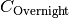) of the grid measures (lines and transformers) are calculated as: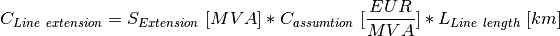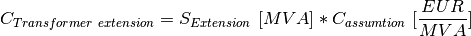The total overnight grid extension costs are given by: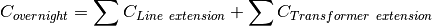The conversion of the given annuity costs of eTraGo is done in etrago_convert_overnight_cost().

### Annuity costs¶

The annuity costs represents project investment costs with an interest as present value of an annuity. The investment years T and the interest rate p are defined as default in eGo with an interest rate () of 0.05 and a number of investment years (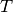) of 40 years. The values are based on the [StromNEV_A1] for the grid investment regulation in Germany.

The present value of an annuity (PVA) is calculated as: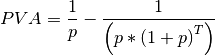In order to calculate the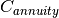of a given period less than a year the annuity costs are factorized by the hours of theand the defined calculation period.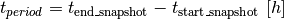The annuity costs () is calculated as: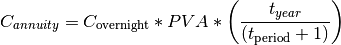### Investment costs ehv/hv¶

The investment costs of the grid and storage expansion are taken from the studies [NEP2015a] for the extra and high voltage components and the [Dena]. The given costs are transformed in respect to PyPSA [€/MVA] format [PyPSA] components for the optimisation.

Overview of grid cost assumtions:

The table displays the transformer and line costs which are used for the calculation with eTraGo.

Table 1 Overview of grid cost assumtions
voltage level component capital costs unit source
110 AC overhead transmission lines 230 EUR/MVA Dena 2012
220 AC overhead transmission lines 290 EUR/MVA NEP 2015
380 AC overhead transmission lines 85 EUR/MVA NEP 2015
DC DC overhead transmission lines 375 EUR/MVA NEP 2015
110/220 transformer 7500 EUR/MVA Dena 2012
110/380 transformer 17333 EUR/MVA NEP 2015
220/380 transformer 14166 EUR/MVA NEP 2015

The eTraGo calculation of the annuity costs per simulation period is defined in set_line_costs() and set_trafo_costs().

Overview of storage cost assumtions:

### Investment costs mv/lv¶

The tool eDisGO is calculating all grid expansion measures as capital or overnight costs. In order to get the annuity costs of eDisGo’s optimisation results the function edisgo_convert_capital_costs() is used. The cost assumption of [eDisGo] are taken from the [Dena] and [CONSENTEC] study. Based on the component the costs including earthwork costs can depend on population density according to [Dena].